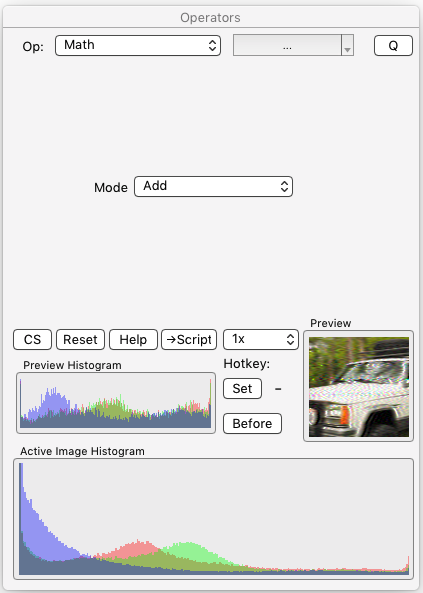Operators Dialog / Operator Details

### § 12.11.57 - Math

Scripting command: math [Mode=0...7]

Note: All available scripting operations are listed on the Script operator page.

This is a compose operation; obeys the  Mask/Alpha setting, and works with the Blending dialog. Brings the origin image (set with o) into the action image according to the mode. Each of the action image's RGB channels are calculated independently against the origin image's RGB channels.

Tip: The origin image must be exactly the same size as the action image.

Add    Adds the origin image to the action image as a signed set of values, where 32768 is zero, below that is progressively more negative, and above that is positive.

Absolute Add    Adds the origin image to the action image as an unsigned set of values, where 0 is zero, and above that is positive.

Subtract    Subtracts the origin image from the action image as a signed set of values, where 32768 is zero, below that is progressively more negative, and above that is positive.

Absolute Subtract    Subtracts the origin image from the action image as an unsigned set of values, where 0 is zero, and above that is positive.

Multiply    Multiplies the action image by the origin image, where the origin image is treated as three channels of a range of zero to one corresponding to the values zero to 65535.

Divide    Divides the action image by the origin image, where the origin image is treated as three channels of a range of zero to one corresponding to the values zero to 65535. Zero value channels are replaced with a value of one to prevent division by zero.

Sine    Multipies the action image by the sine of the origin image, where the origin image is treated as three channels of a range of zero to 360 degrees (or two pi if you're a fan of radians.)

Cosine    Multipies the action image by the cosine of the origin image, where the origin image is treated as three channels of a range of zero to 360 degrees (or two pi if you're a fan of radians.)The Math Dialog